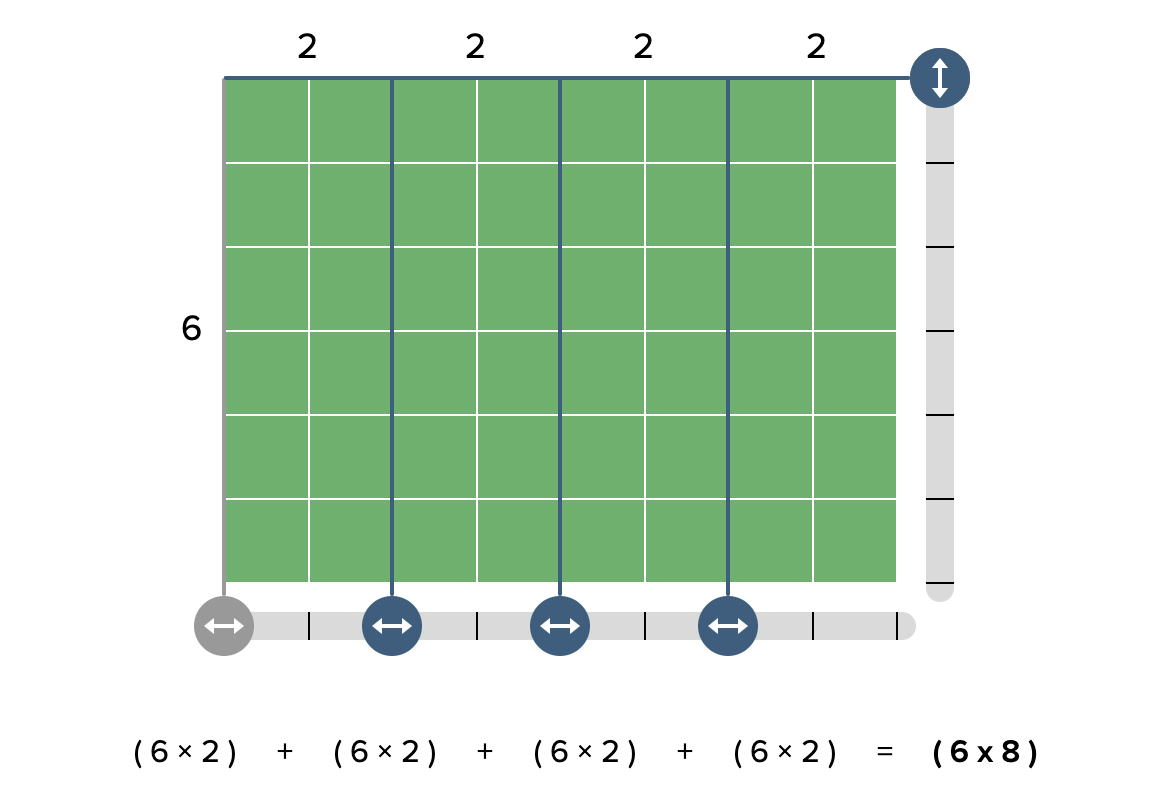# Virtual Manipulatives

 Mathigon PolypadExplore Geometry topics like symmetry, design, shapes, and angles, with polygons, tangrams, kolam tiles, protractors, etc  Explore Number topics like factors/multiples, place values, multiplication, number systems with prime factor circles (like a virtual Prime Climb!), number tiles, adjustable number lines, exploding dots, multiplication grids, etc.  Explore Fractions topics like equivalent fractions and with fraction bars and fraction circles Explore Algebra topics like equality, isolating the variable, combining like terms, multiplying polynomials, with area models, algebra tiles and balance scales. Use the Randomizer to spin, roll, and flip virtual uniform spinners (divided into equally-sized sections), customizable spinners, dice, and "coins" to explore Probability topics.Partial Product Finder by The Math Learning CenterMost of us have had students who have not yet developed fluency with multiplication facts. For most of those students, the challenge is that have been taught to think of the 12 by 12 multiplication table as 144 discrete math facts to memorize. If a student doesn't know 6x8, we want them to be be able to build using groupings they do know. For example, if I don't know 6x8, but I do know 6x2, I can use that 6x2. The Partial Product Finder helps students create visual models for breaking up numbers and using partial products. Ideal for students to explore creating area models for multiplication up to 50x50. (For an introduction, visit Using Partial Products with adult learners).The Math Learning Center Visual Models and Manipulatives: The highlight for me is the interactive, virtual Geoboard, great for exploring area/perimeter, fractions, symmetry, angles, triangles, similarity, rigid transformation, dilations, visual patterns and more.  Other visual models and manipulatives available include number pieces (good for place value), number lines, number frames, coins, pattern shapes (great for fractions), and more.Math Manipulative Collection from Toy TheaterIncludes virtual 100 charts, cubes (great for exploring volume), spinners, dice, Area/Perimeter explorer, geoboards, marble jar (great for estimation & probability), fraction/decimal/percentage strips, a thermometer (great for exploring conversions) and more.